14 Coordinates and Graphics
Weve used ListPlot and ListLinePlot to plot lists of values, where each value appears after the one before. But by giving lists containing pairs of coordinates instead of single values, we can use these functions to plot points at arbitrary positions.
 In:=Out=In:=Out=Generate a sequence of random {x, y} coordinates:
 In:=Out=Another way to get random coordinates:
 In:=Out=In:=Out=We can use coordinates to construct graphics. Earlier we saw how to make graphics of one circle. To make graphics of more than one circle we have to say where each circle is, which we can do by giving the coordinates of their centers.
 In:=Out=If we apply color styles its easier to see which circle is which:
 In:=Out=Make a graphic with 100 randomly placed circles, each with center coordinates up to 50:
 In:=Out=In:=Out=Circle[{x,y}] means a circle centered at position {x,y}. If you dont say otherwise, the circle is given radius 1. You can make a circle of any radius using Circle[{x,y},r].
Use different radii for different circles:
 In:=Out=Make 10 concentric circles:
 In:=Out=Draw larger circles with centers that shift progressively to the right:
 In:=Out=In:=Out=RegularPolygon works much the same as Circle and Disk, except that in addition to giving the position of the center and the size, you also have to specify how many sides the polygon should have.
Make graphics of a size-1 regular pentagon and a size-0.5 regular heptagon:
 In:=Out=You can mix different kinds of graphics objects:
 In:=Out=To make arbitrary graphics, you need the basic graphics primitives Point, Line and Polygon. Point[{x,y}] represents a point at coordinate position {x,y}. To get multiple points, you can either give a list of Point[{x,y}]s, or you can give a list of coordinate positions inside a single Point.
Graphics of three points at specified positions:
 In:=Out=In:=Out=In:=Out=In:=Out=RegularPolygon makes a regular polygon in which all sides and angles are the same. Polygon lets you make any polygon, even strange ones that fold over themselves.
A polygon with 20 corners at random coordinates below 100; the polygon folds over itself:
 In:=Out=Two spheres stacked on top of each other:
 In:=Out=A 3D array of spheres (radius 1/2 makes them just touch):
 In:=Out=A 3D array of points:
 In:=Out=In:=Out=Specify opacity 0.5 for all spheres:
 In:=Out=You can use Manipulate to make graphicsin 2D or 3Dthat can be manipulated.
Manipulate the position and opacity of the second sphere:
 In:=Out=Point[{x,y}] a point at coordinates {x, y} Line[{{1,1},{2,4},{1,2}}] a line connecting specified coordinates Circle[{x,y}] a circle with center at {x, y} Circle[{x,y},r] a circle with center at {x, y} and radius r RegularPolygon[{x,y},s,n] a regular polygon with center {x, y}and n sides each s long Polygon[{{1,1},{2,4},{1,2}}] a polygon with the specified corners Sphere[{x,y,z}] a sphere with center at {x, y, z} Sphere[{x,y,z},r] a sphere with center at {x, y, z} and radius r Opacity[level] specify an opacity level (0: transparent; 1: solid)
14.1Make graphics of 5 concentric circles centered at {0, 0} with radii 1, 2, ... , 5. »
Expected output:
 Out[]=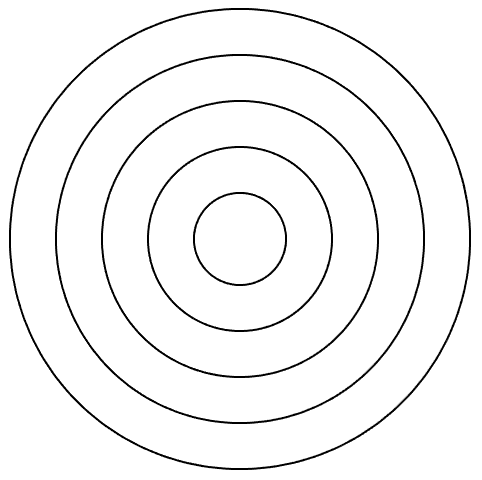Answer & check your solution
14.2Make 10 concentric circles with random colors. »
Sample expected output:
 Out[]=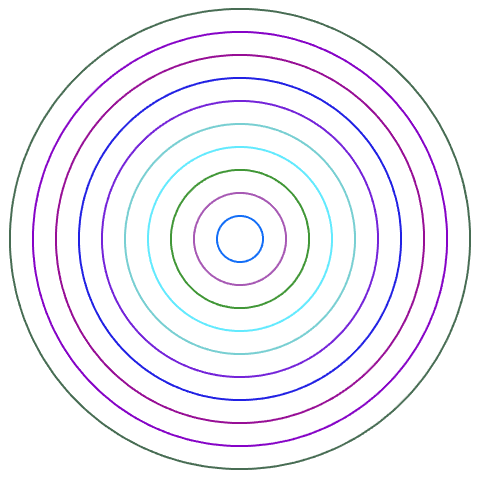Answer & check your solution
14.3Make graphics of a 10×10 grid of circles with radius 1 centered at integer points {x, y}»
Expected output:
 Out[]=Answer & check your solution
14.4Make a 10×10 grid of points with coordinates at integer positions up to 10. »
Expected output:
 Out[]=Answer & check your solution
14.5Make a Manipulate with between 1 and 20 concentric circles. »
Sample expected output:
 Out[]=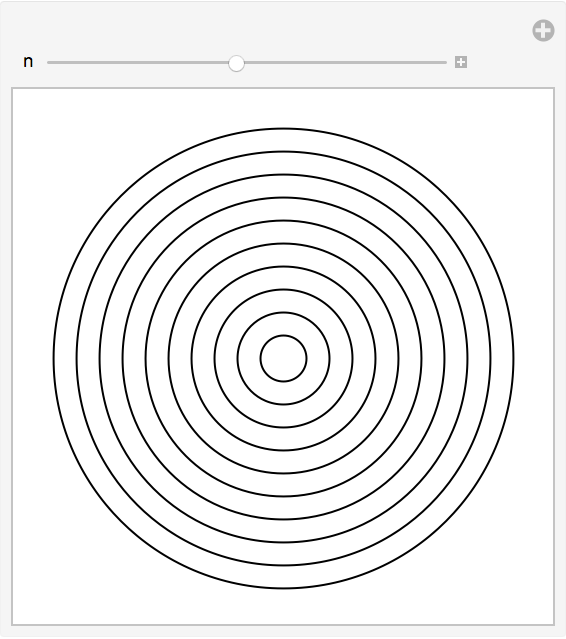Answer & check your solution
14.6Place 50 spheres with random colors at random integer coordinates up to 10. »
Sample expected output:
 Out[]=Answer & check your solution
Expected output:
 Out[]=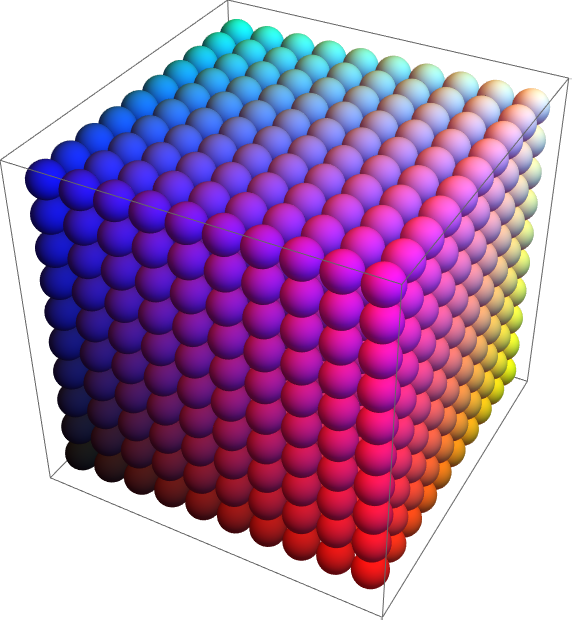Answer & check your solution
14.8Make a Manipulate with t varying between 2 and +2 that contains circles of radius x centered at {t*x, 0} with x going from 1 to 10. »
Sample expected output:
 Out[]=Answer & check your solution
14.9Make a 5×5 array of regular hexagons with size 1/2, centered at integer points. »
Expected output:
 Out[]=Answer & check your solution
Sample expected output:
 Out[]=Answer & check your solution
+14.1Make a Manipulate to create an n×n regular grid of points at integer positions, with n going from 5 to 20. »
Sample expected output:
 Out[]=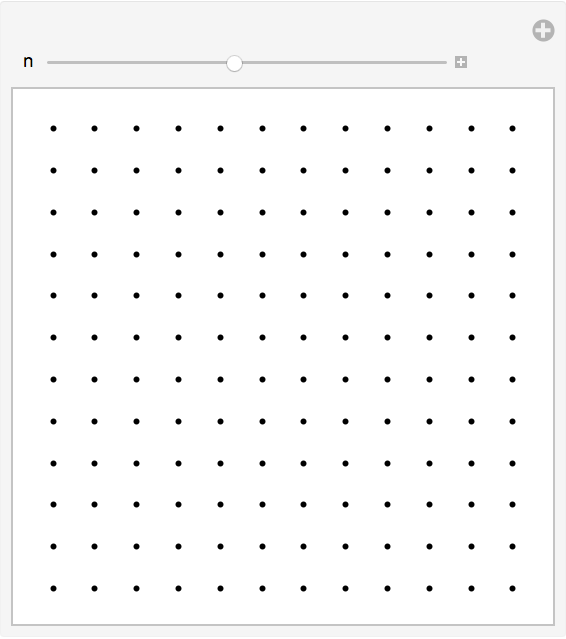Answer & check your solution
+14.2Place 30 radius-1 circles with random colors at random integer coordinates up to 10. »
Sample expected output:
 Out[]=Answer & check your solution
+14.3Display 100 polygons with side length 10, opacity .5, and random choices of colors, sides between 3 and 8, and integer coordinates up to 100. »
Sample expected output:
 Out[]=Answer & check your solution
+14.4Make a 10×10×10 array of points in 3D, with each point having a random color. »
Sample expected output:
 Out[]=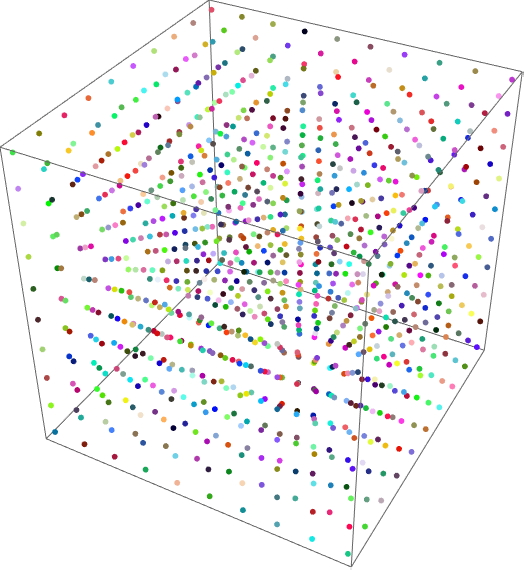Answer & check your solution
+14.5Take the first two digits of numbers from 10 to 100 and draw a line that uses them as coordinates. »
Expected output:
 Out[]=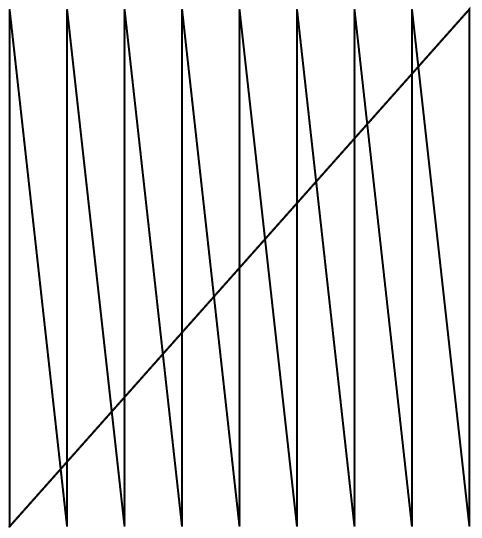Answer & check your solution
+14.6Take the first 3 digits of numbers from 100 to 1000 and draw a line in 3D that uses them as coordinates. »
Expected output:
 Out[]=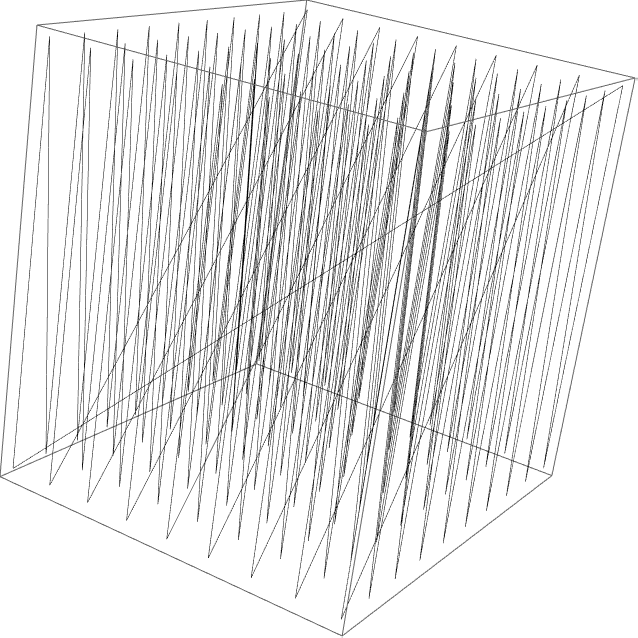Answer & check your solution
What determines the range of coordinates shown?
How can I put axes on graphics?
How do I change the appearance of the edges of a polygon or disk?
What other graphics constructs are there?
Quite a few. Examples include Text (for placing text inside graphics), Arrow (for putting arrowheads on lines, etc.), Inset (for putting graphics inside graphics) and FilledCurve.
How do I get rid of the box around 3D graphics?